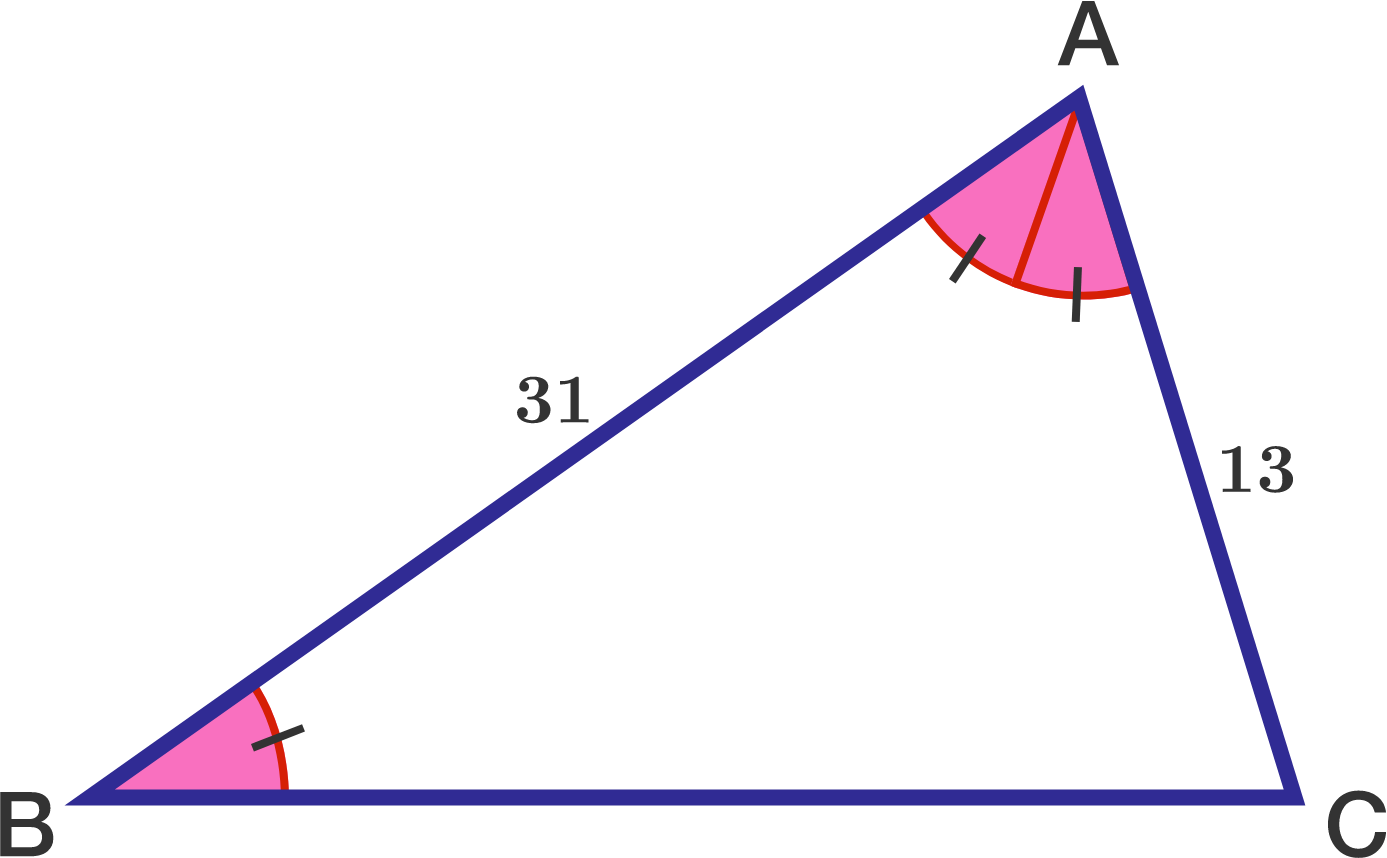# One Angle Twice the Other

Geometry Level 2

In triangle $ABC$, $\angle BAC = 2 \angle ABC$, $AB= 31, AC = 13$. What is $BC^2$?×